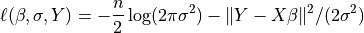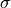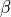Note

This page is a reference documentation. It only explains the class signature, and not how to use it. Please refer to the user guide for the big picture.

3.6.2. nistats.regression.ARModel¶

class nistats.regression.ARModel(design, rho)

A regression model with an AR(p) covariance structure.

In terms of a LikelihoodModel, the parameters are beta, the usual regression parameters, and sigma, a scalar nuisance parameter that shows up as multiplier in front of the AR(p) covariance.

__init__(self, design, rho)

Initialize AR model instance

Parameters
designndarray

2D array with design matrix

rhoint or array-like

If int, gives order of model, and initializes rho to zeros. If ndarray, gives initial estimate of rho. Be careful as ARModel(X, 1) != ARModel(X, 1.0).

fit(self, Y)

Fit model to data Y

Full fit of the model including estimate of covariance matrix, (whitened) residuals and scale.

Parameters
Yarray-like

The dependent variable for the Least Squares problem.

Returns
fitRegressionResults
logL(self, beta, Y, nuisance=None)

Returns the value of the loglikelihood function at beta.

Given the whitened design matrix, the loglikelihood is evaluated at the parameter vector, beta, for the dependent variable, Y and the nuisance parameter, sigma.

Parameters
betandarray

The parameter estimates. Must be of length df_model.

Yndarray

The dependent variable

nuisancedict, optional

A dict with key ‘sigma’, which is an optional estimate of sigma. If None, defaults to its maximum likelihood estimate (with beta fixed) as sum((Y - X*beta)**2) / n, where n=Y.shape, X=self.design.

Returns
loglffloat

The value of the loglikelihood function.

Notes

The log-Likelihood Function is defined asThe parameterabove is what is sometimes referred to as a nuisance parameter. That is, the likelihood is considered as a function of, but to evaluate it, a value ofis needed.

Ifis not provided, then its maximum likelihood estimate:is plugged in. This likelihood is now a function of onlyand is technically referred to as a profile-likelihood.

References

1
1. Green. “Econometric Analysis,” 5th ed., Pearson, 2003.

whiten(self, X)

Whiten a series of columns according to AR(p) covariance structure

Parameters
Xarray-like of shape (n_features)

array to whiten

Returns
whitened_Xndarray

X whitened with order self.order AR